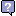English Malay Thai

Flow Rate [volume] Conversion

 Convert what quantity?From: acre foot/day acre foot/hour acre foot/minute acre foot/second acre foot/day [survey] acre foot/hour [survey] acre foot/minute [survey] acre foot/second [survey] acre inch/day acre inch/hour acre inch/minute acre inch/second acre inch/day [survey] acre inch/hour [survey] acre inch/minute [survey] acre inch/second [survey] barrel/day [petroleum] barrel/hour [petroleum] barrel/minute [petroleum] barrel/second [petroleum] barrel/day [UK] barrel/hour [UK] barrel/minute [UK] barrel/second [UK] barrel/day [US] barrel/hour [US] barrel/minute [US] barrel/second [US] barrel/day [US beer/wine] barrel/hour [US beer/wine] barrel/minute [US beer/wine] barrel/second [US beer/wine] billion cubic foot/day billion cubic foot/hour billion cubic foot/minute billion cubic foot/second centiliter/day centiliter/hour centiliter/minute centiliter/second cubem/day cubem/hour cubem/minute cubem/second cubic centimeter/day cubic centimeter/hour cubic centimeter/minute cubic centimeter/second cubic decimeter/day cubic decimeter/hour cubic decimeter/minute cubic decimeter/second cubic dekameter/day cubic dekameter/hour cubic dekameter/minute cubic dekameter/second cubic foot/day cubic foot/hour cubic foot/minute cubic foot/second cubic inch/day cubic inch/hour cubic inch/minute cubic inch/second cubic kilometer/day cubic kilometer/hour cubic kilometer/minute cubic kilometer/second cubic meter/day cubic meter/hour cubic meter/minute cubic meter/second cubic mile/day cubic mile/hour cubic mile/minute cubic mile/second cubic millimeter/day cubic millimeter/hour cubic millimeter/minute cubic millimeter/second cubic yard/day cubic yard/hour cubic yard/minute cubic yard/second cusec deciliter/day deciliter/hour deciliter/minute deciliter/second dekaliter/day dekaliter/hour dekaliter/minute dekaliter/second gallon/day [UK] gallon/hour [UK] gallon/minute [UK] gallon/second [UK] gallon/day [US] gallon/hour [US] gallon/minute [US] gallon/second [US] hectare meter/day hectare meter/hour hectare meter/minute hectare meter/second hectoliter/day hectoliter/hour hectoliter/minute hectoliter/second kiloliter/day kiloliter/hour kiloliter/minute kiloliter/second lambda/day lambda/hour lambda/minute lambda/second liter/day liter/hour liter/minute liter/second milliliter/day milliliter/hour milliliter/minute milliliter/second million acre foot/day million acre foot/hour million acre foot/minute million acre foot/second million cubic foot/day million cubic foot/hour million cubic foot/minute million cubic foot/second million gallon/day [UK] million gallon/hour [UK] million gallon/minute [UK] million gallon/second [UK] million gallon/day [US] million gallon/hour [US] million gallon/minute [US] million gallon/second [US] miner's inch [AZ, CA, OR] miner's inch [CO] miner's inch [ID, WA, NM] ounce/day [UK] ounce/hour [UK] ounce/minute [UK] ounce/second [UK] ounce/day [US] ounce/hour [US] ounce/minute [US] ounce/second [US] petrograd standard/day petrograd standard/hour petrograd standard/minute petrograd standard/second stere/day stere/hour stere/minute stere/second thousand cubic foot/day thousand cubic foot/hour thousand cubic foot/minute thousand cubic foot/second trillion cubic foot/day trillion cubic foot/hour trillion cubic foot/minute trillion cubic foot/second To: acre foot/day acre foot/hour acre foot/minute acre foot/second acre foot/day [survey] acre foot/hour [survey] acre foot/minute [survey] acre foot/second [survey] acre inch/day acre inch/hour acre inch/minute acre inch/second acre inch/day [survey] acre inch/hour [survey] acre inch/minute [survey] acre inch/second [survey] barrel/day [petroleum] barrel/hour [petroleum] barrel/minute [petroleum] barrel/second [petroleum] barrel/day [UK] barrel/hour [UK] barrel/minute [UK] barrel/second [UK] barrel/day [US] barrel/hour [US] barrel/minute [US] barrel/second [US] barrel/day [US beer/wine] barrel/hour [US beer/wine] barrel/minute [US beer/wine] barrel/second [US beer/wine] billion cubic foot/day billion cubic foot/hour billion cubic foot/minute billion cubic foot/second centiliter/day centiliter/hour centiliter/minute centiliter/second cubem/day cubem/hour cubem/minute cubem/second cubic centimeter/day cubic centimeter/hour cubic centimeter/minute cubic centimeter/second cubic decimeter/day cubic decimeter/hour cubic decimeter/minute cubic decimeter/second cubic dekameter/day cubic dekameter/hour cubic dekameter/minute cubic dekameter/second cubic foot/day cubic foot/hour cubic foot/minute cubic foot/second cubic inch/day cubic inch/hour cubic inch/minute cubic inch/second cubic kilometer/day cubic kilometer/hour cubic kilometer/minute cubic kilometer/second cubic meter/day cubic meter/hour cubic meter/minute cubic meter/second cubic mile/day cubic mile/hour cubic mile/minute cubic mile/second cubic millimeter/day cubic millimeter/hour cubic millimeter/minute cubic millimeter/second cubic yard/day cubic yard/hour cubic yard/minute cubic yard/second cusec deciliter/day deciliter/hour deciliter/minute deciliter/second dekaliter/day dekaliter/hour dekaliter/minute dekaliter/second gallon/day [UK] gallon/hour [UK] gallon/minute [UK] gallon/second [UK] gallon/day [US] gallon/hour [US] gallon/minute [US] gallon/second [US] hectare meter/day hectare meter/hour hectare meter/minute hectare meter/second hectoliter/day hectoliter/hour hectoliter/minute hectoliter/second kiloliter/day kiloliter/hour kiloliter/minute kiloliter/second lambda/day lambda/hour lambda/minute lambda/second liter/day liter/hour liter/minute liter/second milliliter/day milliliter/hour milliliter/minute milliliter/second million acre foot/day million acre foot/hour million acre foot/minute million acre foot/second million cubic foot/day million cubic foot/hour million cubic foot/minute million cubic foot/second million gallon/day [UK] million gallon/hour [UK] million gallon/minute [UK] million gallon/second [UK] million gallon/day [US] million gallon/hour [US] million gallon/minute [US] million gallon/second [US] miner's inch [AZ, CA, OR] miner's inch [CO] miner's inch [ID, WA, NM] ounce/day [UK] ounce/hour [UK] ounce/minute [UK] ounce/second [UK] ounce/day [US] ounce/hour [US] ounce/minute [US] ounce/second [US] petrograd standard/day petrograd standard/hour petrograd standard/minute petrograd standard/second stere/day stere/hour stere/minute stere/second thousand cubic foot/day thousand cubic foot/hour thousand cubic foot/minute thousand cubic foot/second trillion cubic foot/day trillion cubic foot/hour trillion cubic foot/minute trillion cubic foot/second Result: